Return to Free Library

Return to Number Menu

In this article we will continue our discussion of square roots by delving deeper into the processes of the individual square roots of 2, 3, and 5.

# Square Roots – √2, √3, √5

There are simple geometric concepts regarding the square roots1:

√2 – the diagonal of a square when the sides equal one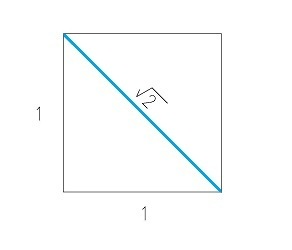√3 – the ratio formed by the Vesica Piscis (the height to width); also relates to the height of a hexagon; also the diagonal of a cube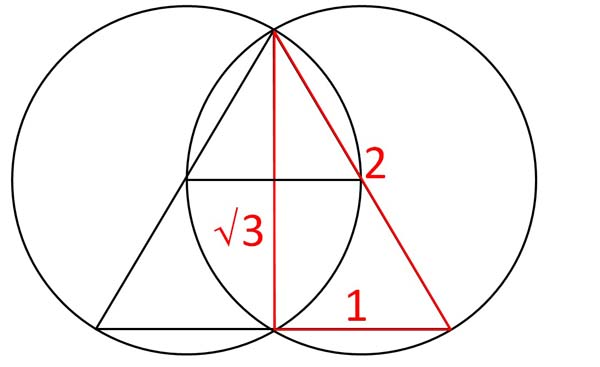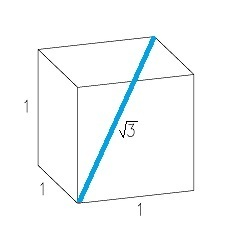√5 – the diagonal of a double square (two squares stacked on top of each other)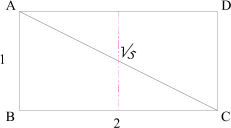# Square Root 2 + Square Root 3 approximates pi

√2 + √3 = π (99.85% accuracy)

π = 3.14159265…

√2 + √3 = 3.14626436…

Remember, the square roots of 2, 3, and 5 all emerge from the Vesica Piscis and corresponding two overlapping circles.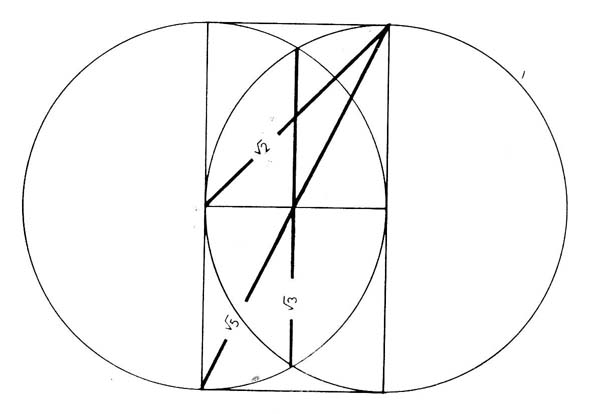We will now begin to explore them one by one.

# √2

The square root of 2 will be explored more fully in the Tetrad (4). We will introduce it here.

√2 = 1.4142135…

The side of a square is called its root (√).

Look at any square. Regardless of its size, its side is its root. For ease of calculation and demonstration we make the side of the square equal to 1.Using the simple Pythagorean theorem: a2 + b2 = c2, we easily see how the diagonal equals √2.

a = 1

b = 1

c = the hypotenuse (diagonal).

So, 12 +12 = c2.

12 = 1 so this makes it:

1 + 1 = c2.

2 = c2.

Therefore, √2 = c.

The square and its diagonal express the creation of Two from Unity (the initial square) and a consequent proliferation of number through a geometric sequence.

Reference Construction Lesson #12: The division of Unity through the drawing of the squares diagonal.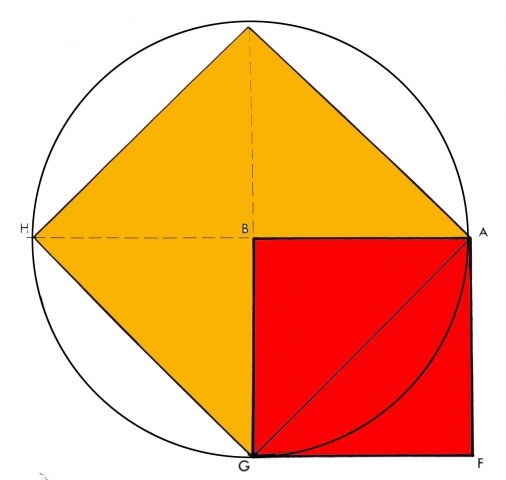The side of the original square, called its ‘root’, is given the value of 1 since it is the first or primary unity. The area of this square is also 1 because 1 x 1 = 1. The simple act of drawing the diagonal has given rise to 2, not because the square has been divided in half, but because square 2 is implied, since the diagonal of square 1 is the root of square 2, and square 2 is exactly double in area to square 1.

Two paradoxes are revealed in the act of its division by the diagonal:

1st Paradox: the coinciding of the two functions of root and diagonal in the geometric moment of the √2. That is, the same line is both root and diagonal. This illustrates the paradox of sameness and difference. One can be the same and different simultaneously.

2nd Paradox: the fact that the half (the square halved by the diagonal) produces the double, as in the generation of musical tone and in the mystery of biological growth from cellular division.

This is the law of the octave. That is, halving = doubling. In this case, halving the square through its diagonal equals a square twice its size. Two grows out of the division of One.

Bruce Rawles writes, “The √2 embodies a profound principle of the whole being more than the sum of its parts.

The orthogonal dimensions (axes at right angles) form the conjugal union of the horizontal and vertical which give birth to the greater offspring of the hypotenuse.

The new generation possesses the capacity for synthesis, growth, integration and reconciliation of polarities by spanning both perspectives equally.

The root of two originating from the square leads to a greater unity, a higher expression of its essential truth, faithful to its lineage.

The fact that the root is irrational expresses the concept that our higher dimensional faculties can’t always necessarily be expressed in lower order dimensional terms.”2# √2 – Unity & Multiplicity

The original square represents Unity. Unity is represented in the finiteness of the square as a wholeness.

Square 2 represents Duality, and the power of proliferation that is multiplicity.

“When one becomes two, we have automatically the potential of endless multiplicity through progression, as we have seen. Thus the extreme, essential polarity of the universe, Unity and Multiplicity, is perfectly represented and observable in the simple drawing of the square and its diagonal.”3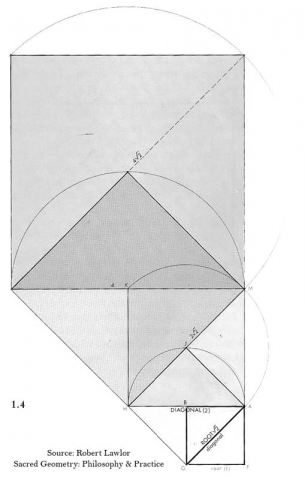# √3 and the Vesica

We discussed the √3 and the Vesica in Articles 21 and 24. We will expand on that information now.

√3 = 1.7320508…

Remember, the relationship of the vertical axis to the horizontal axis of the Vesica Piscis is √3:1.Below is a drawing of the √3 rectangle within the Vesica Piscis.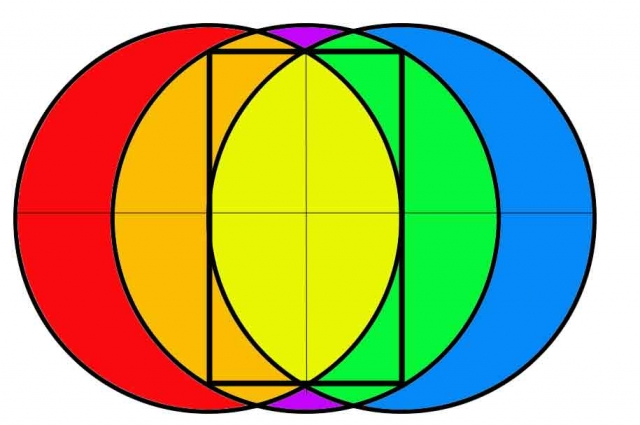Reference Construction Lesson # 13: Proof of the √3 proportion within the Vesica Piscis.

If AB = 1, then DG = 1, CG = 2 and by the Pythagorean Theorem (a2 + b2 =c2) the major axis CD = √(CG2-DG2) = √3.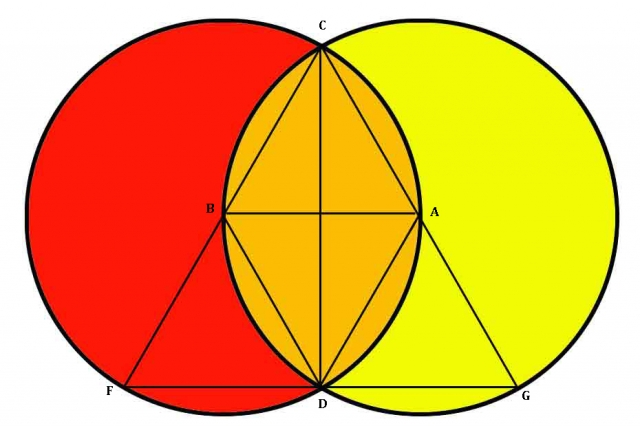In the drawing below the relationship of the smaller axis to the larger axis of the Vesica Piscis in progressive growth visually demonstrates a geometric progression.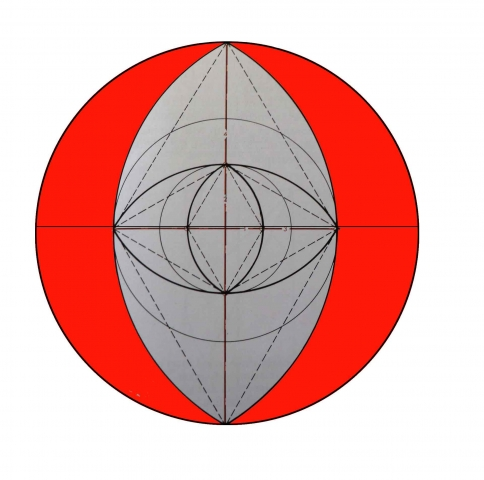Credit: Robert Lawlor, Sacred Geometry: Philosophy & Practice

Now recall that the √3 also represents the height of the hexagon.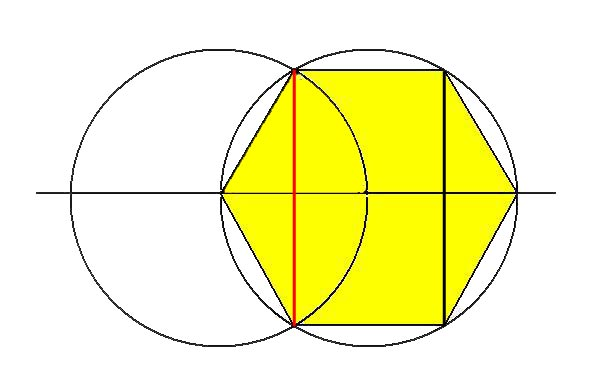“The Piscean Age [represented here by the Vesica] has been characterized as that of the formal embodiment of spirit, manifesting a deeper penetration of spirit into form, with a concurrent deepening of the materialization of spirit: the Word becomes flesh. Thus the √3 is linked to the formative process, and this connection is further clarified when one observes the relationship of the vesica and the √3 to the hexagon, which is the symmetry of order for the measure of the earth, the measure of time (through the 360 degrees of the Great Circle of the heavens) and also the basic formation of mineral crystals, especially of the carbon bonding patterns which allow for the formation of all organic substances.”4

# √3 and the Cube

The cube is the most elementary symbol of the manifest (volumized) formal world.

As we saw above, √3 is the length of the diagonal of a cube.Reference Construction Lesson #14: The √3 and the Cube.

As the division of Unity symbolized by the 2D square yields the √2 function, so the division of Unity symbolized by the cube (representing 3D volume) yields the √3 function.

Diagonal of a square = √2

Diagonal of a cube = √3

“We find that while √2 divides the surface of the square, the √3 divides the volume form of the cube, and we should recall that everything in the created universe is a volume. The formation of any volume structurally requires triangulation, hence the trinity is the creative basis of all form.”5# √5

The √5 (and its related function of phi, the Golden Mean) represent the Regenerative function of life.

√5 = 2.2360679…

Recall from above that the diagonal of a double square rectangle is the √5.The semi-diagonal of a single square = √5/2.

“It seems that the dividing and transforming root powers must be seen at the same time as powers that bind and synthesize, as such principles must often demonstrate two poles of an opposition. The square root of 5 transverses two worlds, indicated by the upper and lower squares, the world of spirit and the world of body.”6

# √5 opens the doorway for the Golden Proportion.

The formula for the Golden Proportion: φ = (1 + √5)/2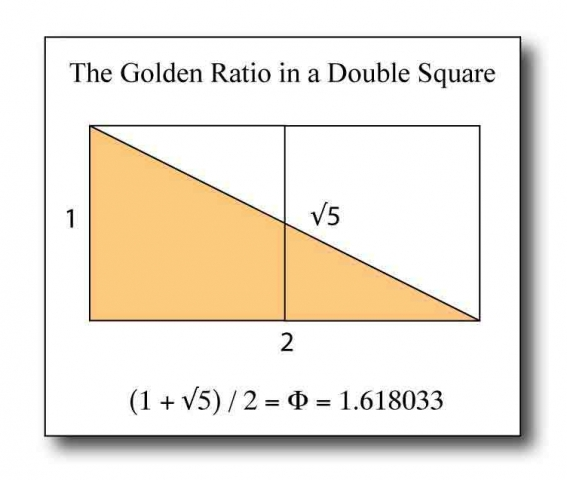Robert Lawlor writes, “The Golden Proportion generates a set of symbols which were used by the Platonic philosophers as a support for the ideal of divine or universal love. It is through the Golden Division that we can contemplate the fact that the Creator planted a regenerative seed which will lift the mortal realms of duality and confusion back towards the image of God.”Reference Construction Lesson #16: The Square Root of 5.

1. http://joedubs.com/square-roots/
2. Rawles, Bruce, Sacred Geometry Tutorial, The Geometry Code, https://www.geometrycode.com/sacred-geometry/
3. Lawlor, Robert, Sacred Geometry: Philosophy & Practice, Thames & Hudson, 1982
4. ibid.
5. ibid.

Return to Free Library

Return to Number Menu